Get instant live expert help with Excel or Google Sheets“My Excelchat expert helped me in less than 20 minutes, saving me what would have been 5 hours of work!”

#### Post your problem and you’ll get Expert help in seconds.

Your message must be at least 40 characters
Our professional Expert are available now. Your privacy is guaranteed.

# How to Use SUMIF Combined with Multiple Criteria

The SUMIF function in Excel is designed for only one criterion or condition.  When we need to sum values based on multiple criteria, we can add two or more SUMIF functions, or we use a combination of SUM and SUMIF functions.  Here’s how.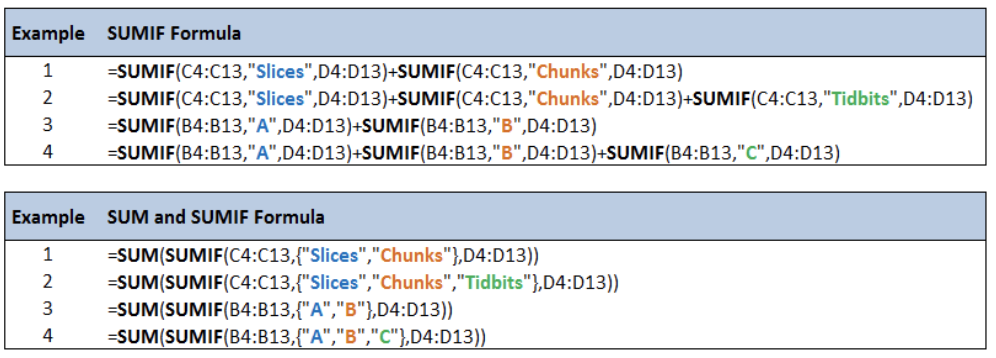Figure 1. SUMIF combined with multiple criteria

## Setting up the Data

Here we have an inventory of stocks for five different products stored in four warehouses.

We want to determine the following:

1. Total stocks for Slices and Chunks
2. Total stocks for Slices, Chunks and Tidbits
3. Total stocks in Warehouse A and B
4. Total stocks in Warehouse A, B and C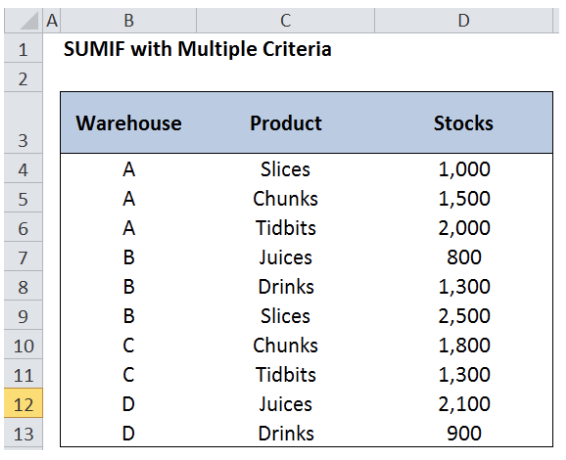Figure 2.  Sample table for SUMIF combined with multiple criteria

## SUMIF function in Excel

SUMIF is a function that sums the values in a specified range, based on one criterion.

### Syntax

`=SUMIF(range,criteria, [sum_range])`

Where

• Range: the data range that will be evaluated using the criteria
• Criteria: the criteria or condition that determines which cells will be added
• Sum_range: the cells that will be added; if left blank, “sum_range” = “range” which means that the range of data that will be added is the same range of data evaluated

### Example of SUMIF with one criterion:

Determine the total stocks for Slices.

In cell G4, enter the formula: =SUMIF(C4:C13,”Slices”,D4:D13)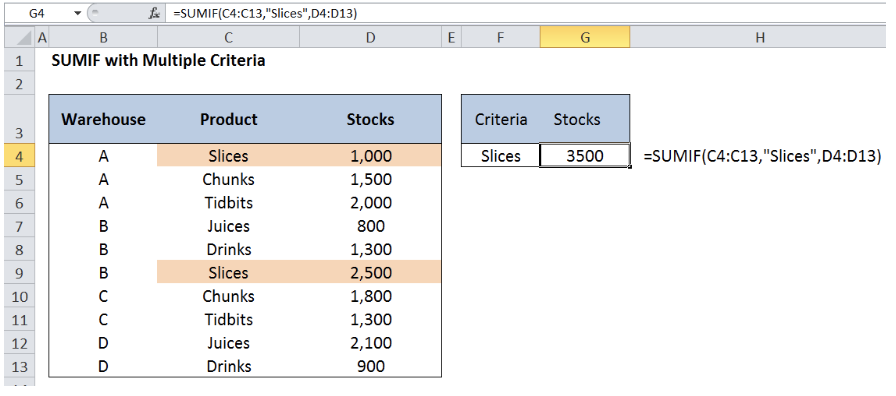Figure 3.  Entering the formula for SUMIF with one criterion

The total stocks for Slices is 3,500: 1,000 in Warehouse A and 2,500 in Warehouse B.

## SUMIF Combined with Multiple Criteria

For multiple OR criteria in the same field, we use several SUMIF functions, one for each category.

### Syntax

`=[SUMIF] + [SUMIF]+...`

`=SUMIF(range1, criteria1, [sum_range1]) + SUMIF(range2, criteria2, [sum_range2])+...`

This formula works like an OR logical formula, which sums values for every criteria that is satisfied.

We want to determine the following:

1. Total stocks for Slices and Chunks
2. Total stocks for Slices, Chunks and Tidbits
3. Total stocks in Warehouse A and B
4. Total stocks in Warehouse A, B and C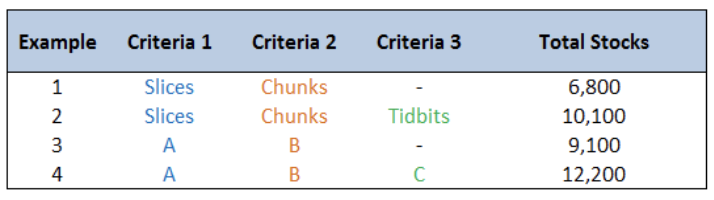Figure 4.  Examples for SUMIF combined with multiple criteria

### Example 1:Total stocks for Slices and Chunks

In cell J4, enter the formula:

`=SUMIF(C4:C13,"Slices",D4:D13)+SUMIF(C4:C13,"Chunks",D4:D13)`

where

• SUMIF(C4:C13,”Slices“,D4:D13) : sum of stocks for Slices
• SUMIF(C4:C13,”Chunks“,D4:D13): sum of stocks for Chunks

The total stocks for Slices and Chunks is 6,800.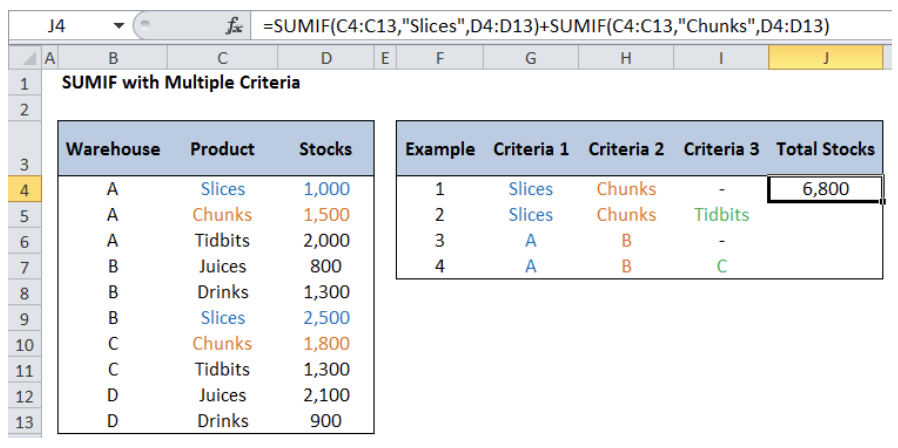Figure 5.  Entering the formula for SUMIF with multiple criteria

### Examples 2 to 4:

For the other examples, enter the formula as shown below: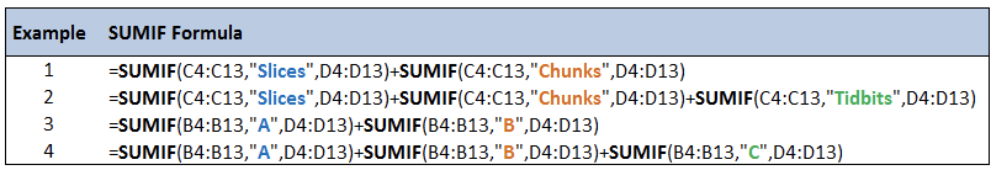Figure 6.  Formula for SUMIF combined with Multiple Criteria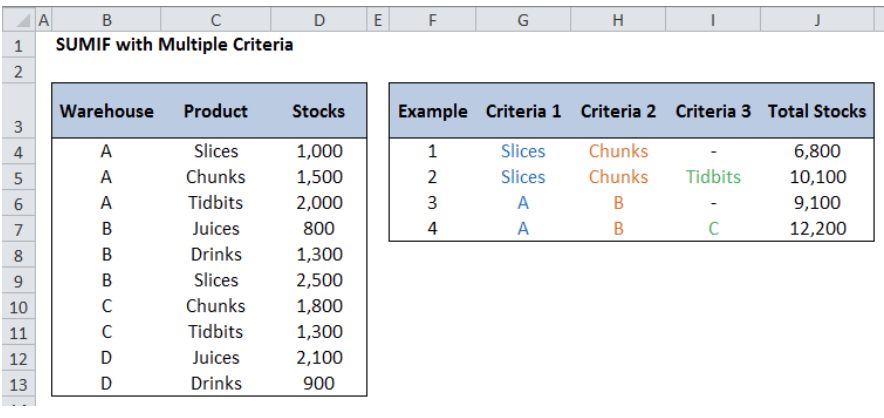Figure 7.  Output for SUMIF combined with Multiple Criteria

We must not get caught up with how many conditions need to be satisfied.  When using SUMIF combined with multiple criteria, we must remember that for each criterion, there must also be one SUMIF function.  This way, we will never be intimidated with any related problem in the future.

## Alternative Formula using SUM and SUMIF

When there are more and more criteria to satisfy, the summation of SUMIF formula will become very long and tedious.  Here is an alternative formula using SUM and SUMIF functions.

### Syntax

`=SUM(SUMIF(range,{criteria1,criteria2,criteria3},sum_range))`

Let’s take the first example where we sum the stocks for “Slices” and “Chunks”.

OLD Formula: “`=``SUMIF(C4:C13,"Slices",D4:D13)+SUMIF(C4:C13,"Chunks``",D4:D13)`

This time, the formula is shorter and simpler:

New Formula: “`=``SUM(SUMIF(C4:C13,{"Slices","Chunks``"},D4:D13))`

Refer to below table and see the difference of the two methods:Figure 8.  Comparison of two methods in using SUMIF combined with multiple criteria

Most of the time, the problem you will need to solve will be more complex than a simple application of a formula or function. If you want to save hours of research and frustration, try our live Excelchat service! Our Excel Experts are available 24/7 to answer any Excel question you may have. We guarantee a connection within 30 seconds and a customized solution within 20 minutes.

### Did this post not answer your question? Get a solution from connecting with the expert.Another blog reader asked this question today on Excelchat:
Solution examplesI am looking for a formula to SUM column L, if Column A matched the relevant date and column C matches the name
Solved by Z. Y. in 16 minsHi, I'm making myself a weekly budget spreadsheet. I've made a sheet with all my monthly outgoings, and I've worked out how to use SUMIF to combine the amounts for each day, but I want to include a reference and don't know how to combine text cells based on a specific date reference... does that make sense?
Solved by E. U. in 60 minsThe formula is: =SUMIF(BAARCH!A:R,summary!A6,BAARCH!P:P) which searches for the name inputted on the summary sheet on the BAARCH worksheet - but I want it to look for the name across a range of worksheets. The column is PP.
Solved by Z. C. in 50 minsI need a formula to pop at the upper left corner of a spreadsheet. If I enter the month "January," I want the column number sum of January =SUM(AB11:AB75) from another section on the same excel page to pop right below the "January" cell, and not display the formula expression, but see the \$100.
Solved by T. Q. in 40 minsCan't add (SUM) in imported numbers from bank account
Solved by F. C. in 40 mins## Subscribe to Excelchat.coAnother blog reader asked this question today on Excelchat: